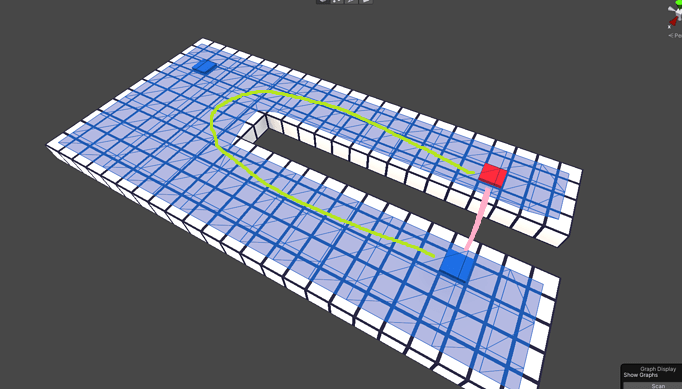# How can I get the graph/path distance between 2 Nodes?

So I’m trying to look through different nodes in the Recast Graph and see which one is further away.

The issue is when distance and path distance don’t match.

In the image above I’m trying to get the graph/path distance from the red node to each blue node, and choose the node that’s closer.

How can I do this? I’ve been able to find and store the graph’s nodes already but I can’t seem to get the graph/path distance, only the default Vector3 distance which is not helpful here.

Hi

Node distance on a recast graph is typically not very useful. Are you sure you don’t want to use a grid graph instead, if you are interested in that?

You might also be interested in MultiTargetPath - A* Pathfinding Project

Hmm I really like the recast graph, though it’s been a while since I changed over so I honestly can’t remember the advantages of the grid graphMultiTargetPath might be worth looking into.

Basically I want an agent to calculate path distance to multiple different places, and then choose whichever returns the shortest route.

Does that sound about right for MultiTargetPath? How many paths can it return? I worry it’ll be too slow, I was hoping to do this without actually calculating each path.

It can return any number of paths. The performance will not be slower than in the worst case searching through every node in the graph. If you would have calculated N paths individually, that would almost surely be slower.

There’s no way to calculate the distance without calculating a path.

But it sounded like you wanted the graph distance in number of nodes, not the world-space distance length of the path, or am I misunderstanding something?

I’ll have to experiment with MultiTargetPaths, thanks for the idea!

Maybe I shouldn’t have considered distance in nodes to be similar to path length in my original post.

Here is a new image:So I want the green line, not the pink line.

The issue is in my real game use case, I wanted to look at dozens or hundreds of possible locations to test that for, not 2 like in this example.

I’ll have to try with MultiTargetPath though because it sounds like it could be a good solution. One origin, multiple end points.

Can I store the end points from the MultiTargetPath on a list? That way I can sort them and find the shortest, longest, random etc.

EDIT: Looking through the example, it looks like `vectorPaths` returns all the info I need, but I find it funny that it returns Vector3s and not Paths. Are those ready to be used or do I still need to do some calculating on them to use one of them as a path?

If you set the `pathsForAll` field to true on the MultiTargetPath it will calculate all paths instead of just the shortest.